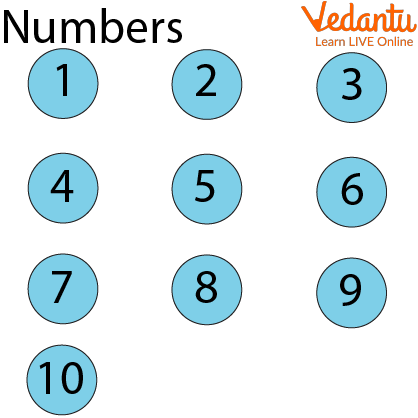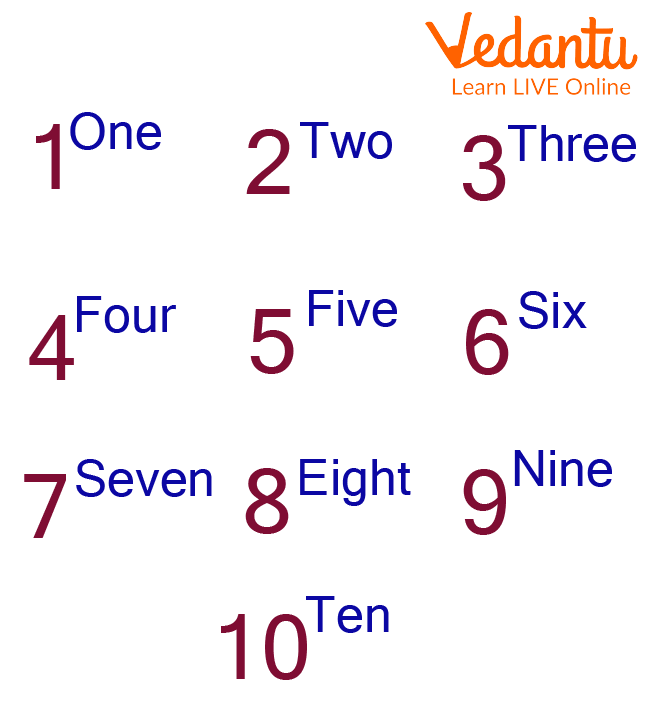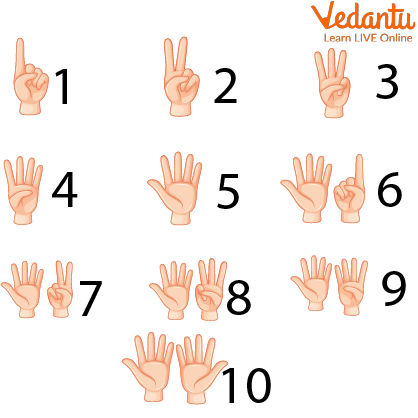Courses
Courses for Kids
Free study material
Free LIVE classes
More

# Counting and Writing Numbers## Introduction to Number Counting

Learning numbers is a fundamental skill that we begin learning in kindergarten. This is also utilised in everyday life. For example, counting the number of students in class, buying a number of chocolates, etc. So you were wondering, what is this number counting? Counting numbers are natural numbers that can be counted. They begin with 1 and continue as 1, 2, 3, 4, and so on. We cannot count zero, hence it is not included in counting numbers. In this tutorial, we will learn more about counting numbers. In this article, we are going to learn about counting and why we need numbers.Numbers

## What is Counting?

The practice of expressing the number of components or objects that are presented is known as counting. Natural numbers that can be numbered and are always positive are included in the category of counting numbers.

## Why Do We Need Numbers?

Numbers are necessary for everyday life because we must count the number of hours, days, money, and so on. Numbers can be numbered and written in words such as one, two, three, and four. They can be counted both forward and backward. We use skip counting, reverse counting, counting by 2s, counting by 5s, and many more techniques to count these numbers.

## Uses of Numbers

Our lives depend heavily on numbers. In order to determine your daily allowance for your food, transport, and other expenses, you can use numbers in your daily life by phoning a friend or family member on your cell phone.

Anything using the concepts of proportion and percentage, such as cooking. weighing produce, meat, poultry, and other items in the market, moving between levels or locations in the building via elevators, examining the cost of things that are on sale in a mall, Inquiring about the Facebook post likes you received, changing the channel of your preferred TV programs, the amount of time you spent at work or in class.Uses of Numbers in Daily Life

## Number Names

A technique to express numbers is using a number name. There are terminologies used to describe numbers. Numbers in words have been taught to students in primary education. These are the cornerstones of mathematics. All students should know by heart the counting name of the numbers. They will be able to accurately spell all the numbers if they learn the names and their spellings. Children should be taught the names of the numbers 1 through 10, then on. All of the counting digits' names ought to be familiar to them. Let's examine the reading and writing of digits 1 through 10.

## Number Names 1 to 10

Let's study numbers and the number system up to ten:Number Names

Each learner must be able to identify the names of the numbers. These fundamental maths concepts will aid students in correctly spelling the numbers. Additionally, students may readily write such numbers when they attend lessons and learn how to do so from their teachers. Mathematics heavily relies on numbers. These numbers serve as the foundation for all mathematical and arithmetic processes, not just in lower-level courses but also in primary education.

## Counting in Numbers with Hands

Using our hands and fingers, we can count from 1 to 10 as well. Visual maths is proven to hasten a child's mathematical development. The physical act of using hands and fingers connects numbers to their symbolic representation. Additionally, using this method will make it easier to recall the names of the numbers being tallied.Counting Numbers with Hands

## Solved Examples

Q1. Write the counting numbers from 1 to 20.

Ans: Counting numbers from 1 to 20 are as follows: 1, 2, 3, 4, 5, 6, 7, 8, 9, 10, 11, 12, 13, 14, 15, 16, 17, 18, 19, 20.

Q2. Write the odd counting numbers less than 9.

Ans: Odd numbers are those numbers that are not divisible by 2. So, the odd counting numbers less than 9 are 1, 3, 5, and 7.

Q3. What are Counting Numbers Less than 10?

Ans: Counting numbers less than 10 can be listed as, 1, 2, 3, 4, 5, 6, 7, 8, 9.

## Practice Problem

Q1. Write the Even Counting Numbers less than 12.

Ans: 2, 4, 6, 8, and 10.

Q2. Write the Odd Counting Numbers Between 6 and 16.

Ans: 7, 9, 11, 13, 15.

## Summary

The term "counting numbers" refers to all natural numbers. These are always positive whole numbers, such as 0, 1, 2, 3, 4, 5, and 6. Mathematical number systems depend heavily on the infinite counting numbers, which could be counted. The fundamental skill we begin learning in kindergarten is how to count. This is employed in daily life as well. Numerological examples can be found everywhere, even in daily life. the number of days in the year, the number of candies delivered to students in class, and so forth.

Last updated date: 25th Sep 2023
Total views: 114.3k
Views today: 4.14k

## FAQs on Counting and Writing Numbers

1.  How do you express a number in words?

When you write a number in words, you must use the alphabet to spell it out. Both words and numbers can be used to express numbers. For instance, 100,000 words are written as 100,000 or 100,000. Based on the place value of the digits, such as ones, tens, hundreds, thousands, and so forth, any natural numbers can be expressed in words. One, two, three, four, five, six, seven, eight, and nine are the words used to express the place value of a number from 1 to 9. The smallest number that is in the tens place is 10, which is represented by the word ten.

2. By the standard form of numbers, what do you mean?

A number can be expressed in a fashion that adheres to specific standards by using its standard form. Standard form refers to any number that may be expressed as a decimal number between 1.0 and 10.0 when multiplied by a power of 10. Writing numbers in their exponent or simplest form when they are too large or too tiny is referred to as a standard form of numbers. The number between 1 and 10 in decimal notation, multiplied by powers of 10, is used to represent it. 9000000, for instance, can be expressed as follows: 9000000 = $9 \times 10^6$

3. What are whole numbers and natural numbers?

Whole numbers start from 0 to infinite whereas natural numbers start from 1 to infinite. The main difference is zero.

4. Explain fractions in brief with a few examples.

The numbers which are in the form $\dfrac{p}{q}$ where q is not equal to 0. Basically, fractions represent parts of a whole number. For example $\dfrac{2}{3}$, $\dfrac{7}{8}$  etc.